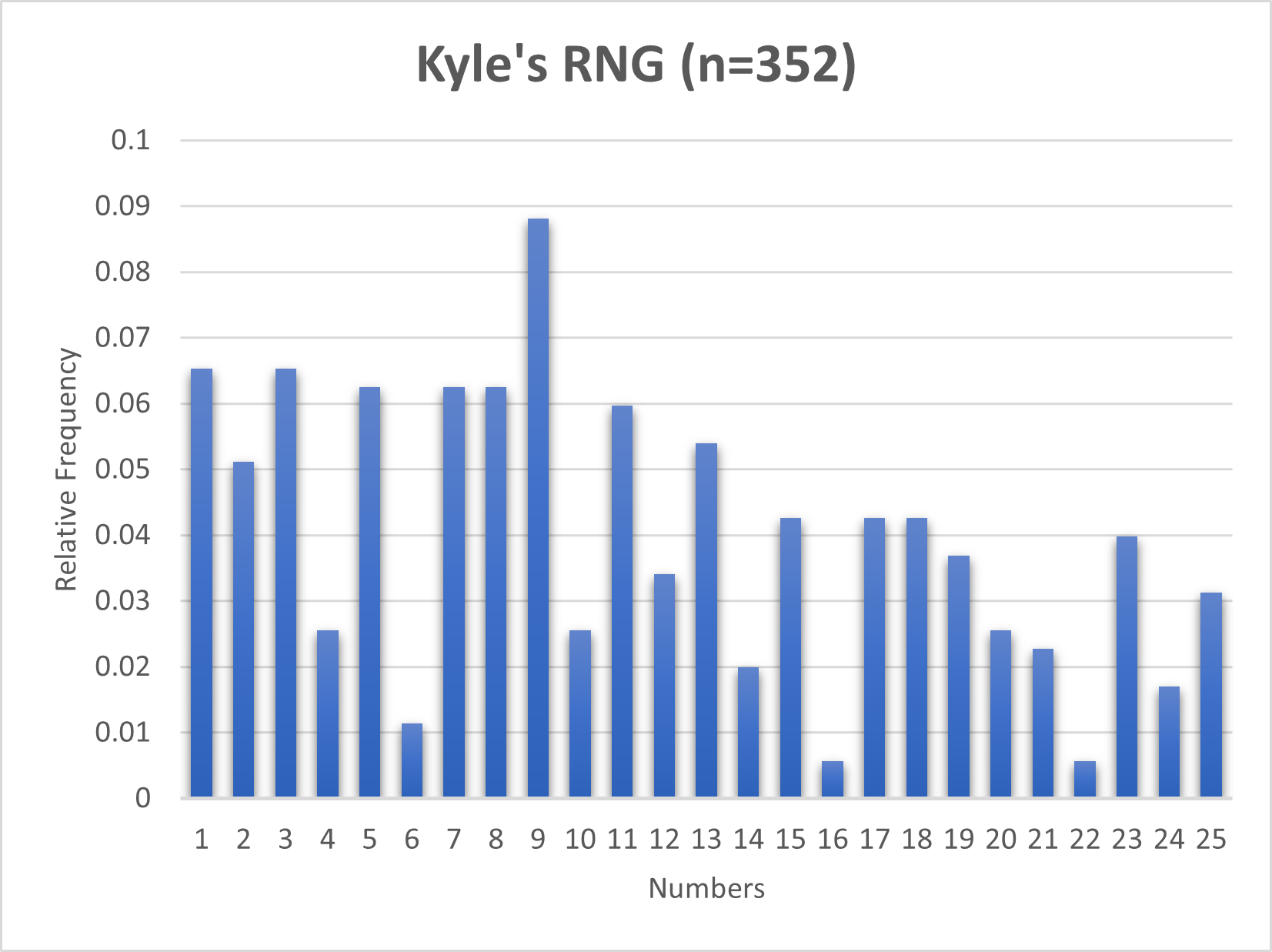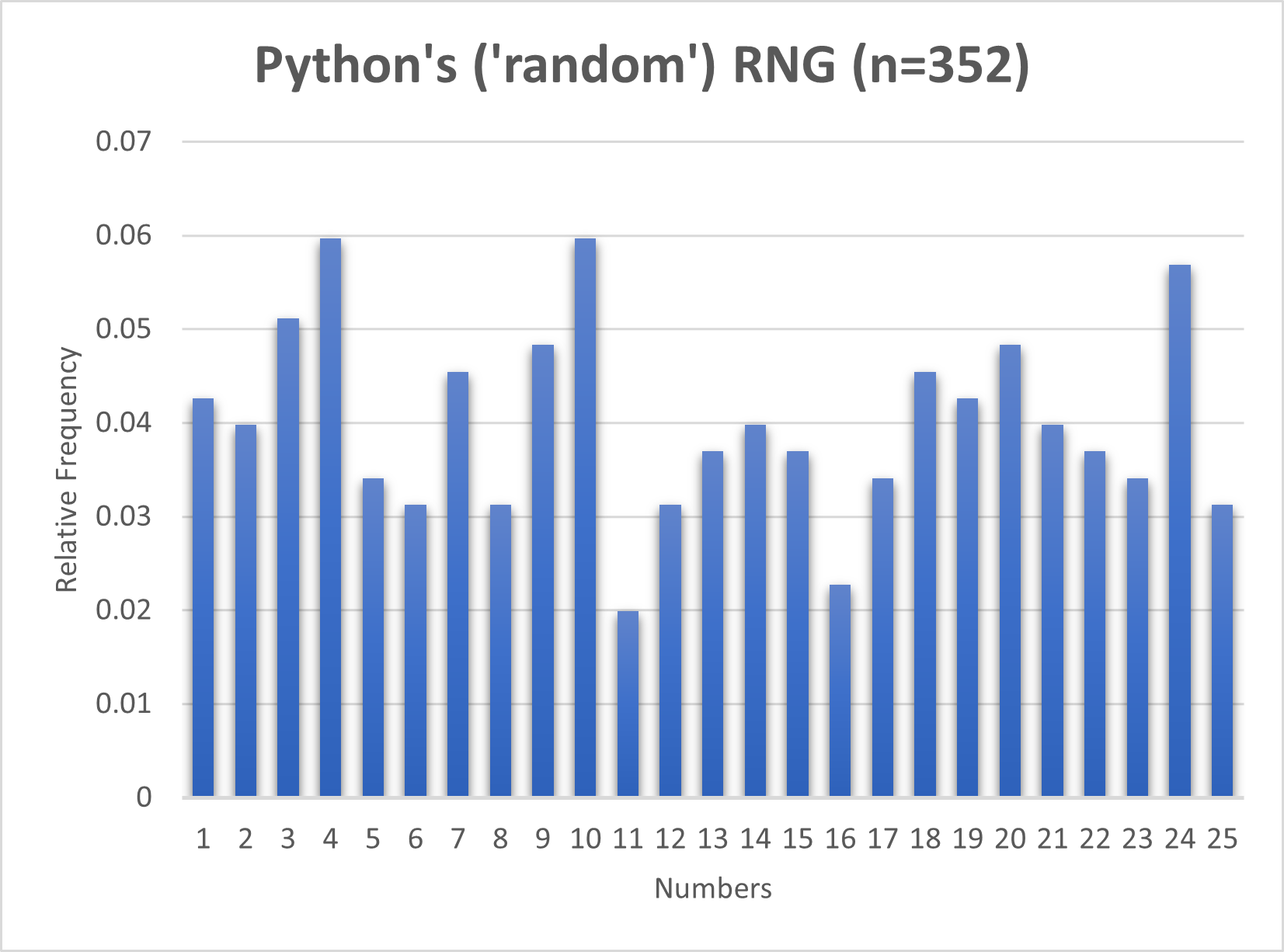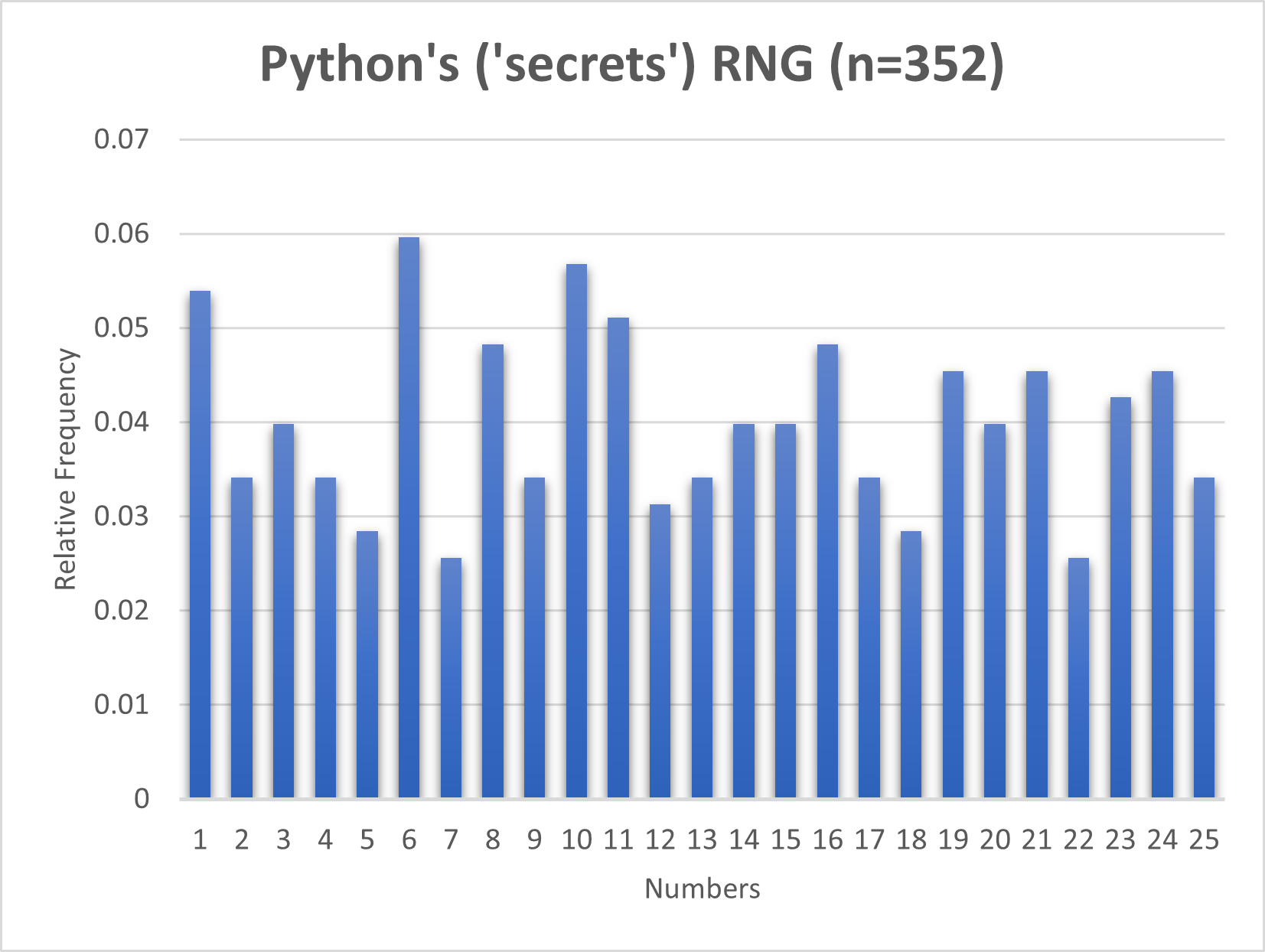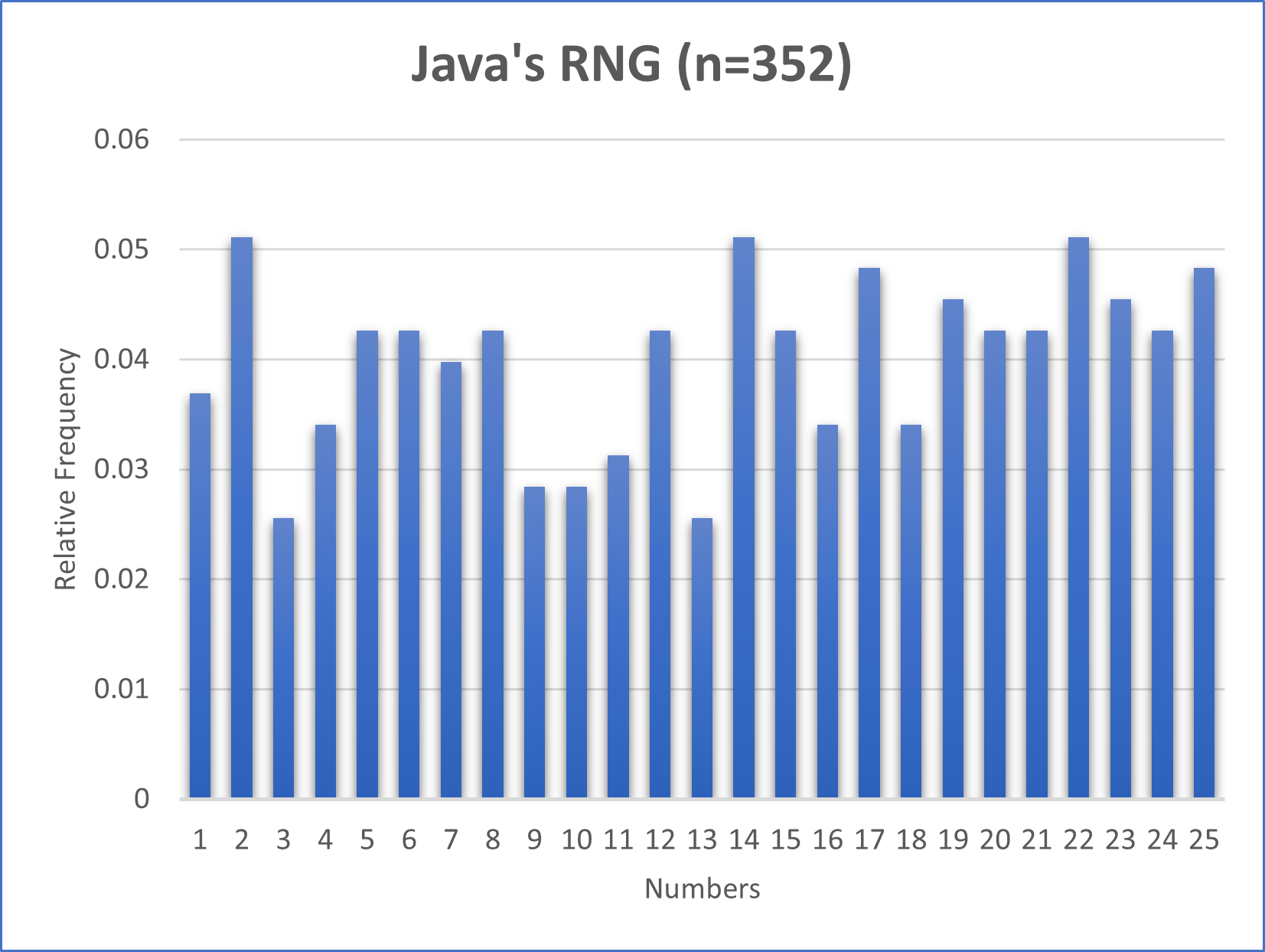# Are you random? How Computers Generate Random Numbers from Non-Random Things### Are you random? How Computers Generate Random Numbers from Non-Random Things

Published May 12, 2021

Updated May 13, 2021

When I ask you for a random number between one and ten and you reply with seven, how can I tell if this was truly a random number? First when most people ask for a random number within some range, they generally mean "give me a number that is chosen with equal probability amongst all other numbers within the range." In other words, they are asking for a number from a uniform probability distribution (as opposed to other probability distributions like the Gaussian or Pareto distributions.) So how can I tell whether or not this single number you've given me comes from a uniform probability distribution? Well, I can't really say anything about it; with only one number I can either accept that it was random or accuse you of having a biases for pushing sevens onto people.

However if I ask you for a sample of one-hundred random numbers between one and ten, I could then infer from this sample if you are indeed choosing numbers from a uniform distribution. There are a few ways to do this. If you've taken a statistics class where you learned about hypothesis testing, you would be aware that there is a mathematical way to say, with some amount of confidence, that a statement is correct (or rather that one statement is rejected in favour of another.) For our purposes we could use a frequency test such as the Chi-Square Test, where we begin by assuming that the numbers you've given me are generated from the uniform distribution, calculate a Chi test-statistic, and see how it matches up against whatever level of confidence we'd like to work at. This comparison will either give me the evidence to reject the assumption, instead favouring that the numbers are not from a uniform distribution, or if it doesn't yield the evidence then I'll be stuck assuming that you are random.

For this post I'll take a more visual but let rigours approach, albeit using the same reasoning. For a sample of a hundred numbers each being a number between one and ten, the "perfect" distribution that matches up to the uniform distribution would count each of the ten numbers exactly ten times. If I were to plot both this distribution and the distribution of numbers you gave me, I could visually determine how much your distribution varied from this perfect uniform distribution and make my decision that way.

## How random am I?

To see how random I was I conducted the following experiment. A few times during the day I would take a minute to write down random numbers between one and twenty-five. I would only write down about ten to at a time to avoid being biased by the previously written numbers. In total I came up with n=352 numbers. Below I have plotted the relative frequency of each number I generated. I decided to compare myself to random number generators that are a part of the Python and Java programming languages and plotted their numbers below (more on them later.) For twenty-five numbers, the uniform distribution would result in every number having a relative frequency of 0.04, or for a sample size of 352 each number should be generated about fourteen times.From my plot it would appear that I have a biases for choosing nine and avoid numbers six, sixteen, and twenty-two. I also seem to favour single digit numbers. Comparing against the other plots, it would appear that I am less random than the programming language's random number generators (note the scaling of the y-axis.) While none of the plots achieve the ideal 0.04 value for each number, they ensure that values fall within the interval [0.02, 0.06] with Java's random number generator having all values falling with [0.025, 0.051].

Perhaps this sample size was too small to adequately capture how random I am, yet humans in general have problems being random. The ability to reason, to see some events as more probable than others, have served us well. Indeed many systems in our society, if not all, function better when we can reduce the probability of random events occurring and live our lives in a deterministic manner. Further each of us are results of our own past experiences. These experiences produce an inherit bias within us that effects man of our actions. For example many people likely know the first twenty-five numbers by the age of seven or eight and only use the first hundred numbers in school. With sufficient time (and sample size) it would be interesting to preform a similar experiment using the first 1000 numbers and see if there is a bias towards generating more familiar numbers. These difficulties lead to an interesting problem. If humans are poor at generating random numbers, how can we expect a computer, a tool the only does what it is told, generate random numbers?

## Pseudorandom Numbers

Computers cannot be random. Sure, we've all experienced times when despite our wishes computers have returned seemingly random search results, but these are all well-defined instructions. When computers yield unexpected results these are typically the result of either poor design and/or implementation by whoever programmed the software. At its core a computer continuously executes whatever well-defined instructions it is presented with.

Yet the results of these instructions can appear random to many. A sequence of numbers that can appear random are called pseudorandom. The prefix of pseduo- is used since if the underlying mechanics of how the sequence is generated are known then the sequence can be perfectly predicted. Here is a simple way to generate pseudorandom numbers. We start with some predefined numbers: `a,b,m` and `x. x` will be the first number in our sequence of random numbers (`x` is called the seed of the generation as it start the sequence.) To generate the sequence we update the value of x using the following rule:

`x <- (a*x+b) modulo m,` where module is the integer remainder of `a*x+b` when divided by m.

As an example let x=8, a=6, b=5, and m=13. The next number in the sequence is 1 since` 6*8+5 modulo 13 = 53 modulo  13 = 1`, as `53 / 13 = 4 + (1/13)`. With `x=1` we continue to generate the sequence and end up with the values:

8, 1, 11, 6, 2, 4, 3, 10, 0 5, 9, 7, 8, 1, 11, …

And so on.

Some interesting things to note. The sequence ends up cycling through the same numbers again and again. From the first number there 12 unique numbers between 0 and 11. This is a result of the modulo operator (anything mod n with be in the interval [0,n-1]) and the fact that the chosen value of m=13 was a prime number (a number which is only divisible by 1 and itself.)  Typically m is chosen to be a large prime number with a and b chosen such the spread of numbers in the resulting sequence is random enough for the user. Finally most modern computers employ a more complex version of the above procedure known as the "Merseene Twister Algorithm" due to its usage of a Merseene prime - a prime of the form 2^n-1 for some n and make use of the computer's internal clock when selecting the seed value. Better yet, a computer can maintain several random number generators and use one of them to decide from which generator the next random number should be pulled.

## But Can We Do Better?

A computer many not be able to generate truly random numbers, but it can observe a random process and use these observations to generate a sequence. For example consider a vision system that is watching a six-sided die in a container. Every second a "kick" is applied to the base of the container rolling the die. Through the camera the computer can record which value is rolled and use this value to generated a random number (e.g. as a seed in the above method) or use the value outright.

Observing real-world phenomenon is indeed how systems that use random numbers for import purposes (e.g. security.) generate them. Our current understanding of particle physics - the laws that govern the physics of extremely small particles - are based on several random (stochastic) models that describe how these particles interact with one another. Along with some examples of how random numbers are used, Anton Spraul discusses how computer with hardware for sending and receiving wireless signals can run true random experiments whose results are interpreted as random numbers.

Thanks for reading! And for the statisticians out there, according to graphpad.com, the Chi-square statistic turns out to be 96.722 with 24 degrees of freedom, a p-value of less than 1 /10,000. In other words, I am not "t'lol random xD" as I first thought.

Photo Credits: Alex Chambers